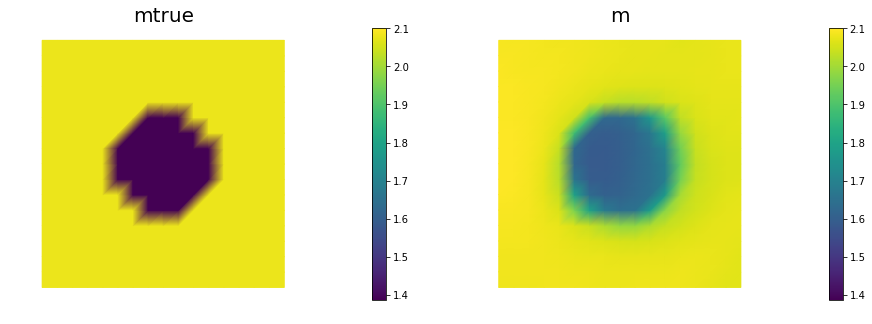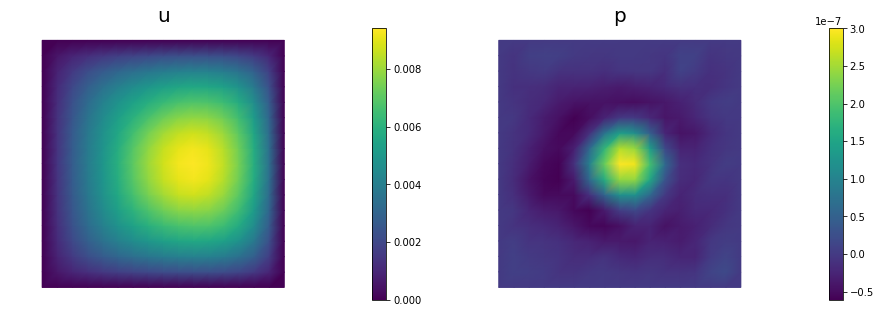# Coefficient field inversion in an elliptic partial differential equation

We consider the estimation of a coefficient in an elliptic partial differential equation as a first model problem. Depending on the interpretation of the unknowns and the type of measurements, this model problem arises, for instance, in inversion for groundwater flow or heat conductivity. It can also be interpreted as finding a membrane with a certain spatially varying stiffness. Let $\Omega\subset\mathbb{R}^n$, $n\in\{1,2,3\}$ be an open, bounded domain and consider the following problem:

where $u$ is the solution of

Here $m \in \mathcal{M}:=\{m\in L^{\infty}(\Omega) \bigcap H^1(\Omega)\}$ denotes the unknown coefficient field, $u \in \mathcal{V}:= H^1_0(\Omega)$ the state variable, $u_d$ the (possibly noisy) data, $f\in H^{-1}(\Omega)$ a given volume force, and $\gamma\ge 0$ the regularization parameter.

### The variational (or weak) form of the state equation:

Find $u\in \mathcal{V}$ such that

where $\mathcal{V} := H_0^1(\Omega)$ is the space of functions vanishing on $\partial\Omega$ with square integrable derivatives.

Above, $(\cdot\,\cdot)$ denotes the $L^2$-inner product, i.e, for scalar functions $u,v$ defined on $\Omega$ we write

and similarly for vector functions $\boldsymbol{u}, \boldsymbol{v}$ defined on $\Omega$ we write

The Lagrangian functional $\mathscr{L}:\mathcal{V}\times\mathcal{M}\times\mathcal{V}\rightarrow \mathbb{R}$ is given by

Then the gradient of the cost functional $\mathcal{J}(m)$ with respect to the parameter $m$ is

where $u \in H_0^1(\Omega)$ is the solution of the forward problem,

and $p \in H_0^1(\Omega)$ is the solution of the adjoint problem,

### Steepest descent method.

Written in abstract form, the steepest descent methods computes an update direction $\hat{m}_k$ in the direction of the negative gradient defined as

where the evaluation of the gradient $\mathcal{G}(m_k)$ involve the solution $u_k$ and $p_k$ of the forward and adjoint problem (respectively) for $m = m_k$.

Then we set $m_{k+1} = m_k + \alpha \hat{m}_k$, where the step length $\alpha$ is chosen to guarantee sufficient descent.

### Goals:

By the end of this notebook, you should be able to:

• solve the forward and adjoint Poisson equations
• understand the inverse method framework
• visualise and understand the results
• modify the problem and code

### Mathematical tools used:

• Finite element method
• Armijo line search

### Import dependencies

from __future__ import print_function, division, absolute_import

import matplotlib.pyplot as plt
%matplotlib inline

import dolfin as dl
from hippylib import nb

import numpy as np
import logging

logging.getLogger('FFC').setLevel(logging.WARNING)
logging.getLogger('UFL').setLevel(logging.WARNING)
dl.set_log_active(False)

np.random.seed(seed=1)


### Model set up:

As in the introduction, the first thing we need to do is to set up the numerical model.

In this cell, we set the mesh mesh, the finite element spaces Vm and Vu corresponding to the parameter space and state/adjoint space, respectively. In particular, we use linear finite elements for the parameter space, and quadratic elements for the state/adjoint space.

The true parameter mtrue is the finite element interpolant of the function

The forcing term f and the boundary conditions u0 for the forward problem are

# create mesh and define function spaces
nx = 32
ny = 32
mesh = dl.UnitSquareMesh(nx, ny)
Vm = dl.FunctionSpace(mesh, 'Lagrange', 1)
Vu = dl.FunctionSpace(mesh, 'Lagrange', 2)

# The true and initial guess for inverted parameter
mtrue = dl.interpolate(dl.Expression('std::log( 8. - 4.*(pow(x - 0.5,2) + pow(x - 0.5,2) < pow(0.2,2) ) )', degree=5), Vm)

# define function for state and adjoint
u = dl.Function(Vu)
m = dl.Function(Vm)
p = dl.Function(Vu)

# define Trial and Test Functions
u_trial, m_trial, p_trial = dl.TrialFunction(Vu), dl.TrialFunction(Vm), dl.TrialFunction(Vu)
u_test,  m_test,  p_test  = dl.TestFunction(Vu),  dl.TestFunction(Vm),  dl.TestFunction(Vu)

# initialize input functions
f  = dl.Constant(1.0)
u0 = dl.Constant(0.0)

# plot
plt.figure(figsize=(15,5))
nb.plot(mesh, subplot_loc=121, mytitle="Mesh", show_axis='on')
nb.plot(mtrue, subplot_loc=122, mytitle="True parameter field")
plt.show()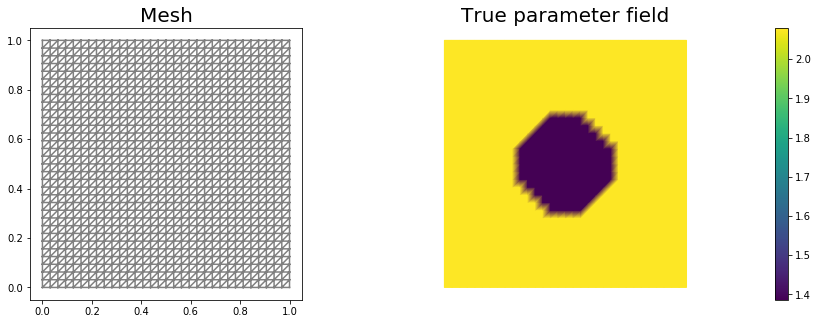# set up dirichlet boundary conditions
def boundary(x,on_boundary):
return on_boundary

bc_state = dl.DirichletBC(Vu, u0, boundary)


### Set up synthetic observations:

To generate the synthetic observation we first solve the PDE for the state variable utrue corresponding to the true parameter mtrue. Specifically, we solve the variational problem

Find $u\in \mathcal{V}$ such that

$\underbrace{(e^{m_{\text true}} \nabla u,\nabla v)}_{\; := \; a_{\rm true}} - \underbrace{(f,v)}_{\; := \;L_{\rm true}} = 0, \text{ for all } v\in \mathcal{V}$.

Then we perturb the true state variable and write the observation ud as

Here the standard variation $\sigma$ is proportional to noise_level.

# noise level
noise_level = 0.01

# weak form for setting up the synthetic observations
L_true = f * u_test * dl.dx

# solve the forward/state problem to generate synthetic observations
A_true, b_true = dl.assemble_system(a_true, L_true, bc_state)

utrue = dl.Function(Vu)
dl.solve(A_true, utrue.vector(), b_true)

ud = dl.Function(Vu)
ud.assign(utrue)

# perturb state solution and create synthetic measurements ud
# ud = u + ||u||/SNR * random.normal
MAX = ud.vector().norm("linf")
noise = dl.Vector()
A_true.init_vector(noise,1)
noise.set_local( noise_level * MAX * np.random.normal(0, 1, len(ud.vector().get_local())) )

ud.vector().axpy(1., noise)

# plot
nb.multi1_plot([utrue, ud], ["State solution with mtrue", "Synthetic observations"])
plt.show()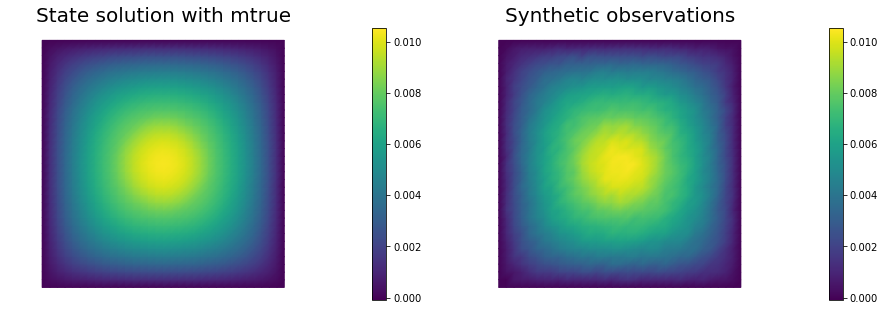### The cost functional evaluation:

# Regularization parameter
gamma = 1e-9

# Define cost function
def cost(u, ud, m, gamma):
misfit = 0.5 * dl.assemble( (u-ud)**2*dl.dx)
return [reg + misfit, misfit, reg]


### Setting up the variational form for the state/adjoint equations and gradient evaluation

Below we define the variational forms that appears in the the state/adjoint equations and gradient evaluations.

Specifically,

• a_state, L_state stand for the bilinear and linear form of the state equation, repectively;
• a_adj, L_adj stand for the bilinear and linear form of the adjoint equation, repectively;
• CTvarf, gradRvarf stand for the contributions to the gradient coming from the PDE and the regularization, respectively.

We also build the mass matrix $M$ that is used to discretize the $L^2(\Omega)$ inner product.

# weak form for setting up the state equation
L_state = f * u_test * dl.dx

# weak form for setting up the adjoint equations
L_adj = - dl.inner(u - ud, p_test) * dl.dx

# Mass matrix in parameter space
Mvarf    = dl.inner(m_trial, m_test) * dl.dx
M = dl.assemble(Mvarf)


### Finite difference check of the gradient

We use a finite difference check to verify that our gradient derivation is correct. Specifically, we consider a function $m_0\in \mathcal{M}$ and we verify that for an arbitrary direction $\tilde{m} \in \mathcal{M}$ we have $r := \left| \frac{ \mathcal{J}(m_0 + \varepsilon \tilde{m}) - \mathcal{J}(m_0)}{\varepsilon} - \mathcal{G}(m_0)(\tilde{m})\right| = \mathcal{O}(\varepsilon).$

In the figure below we show in a loglog scale the value of $r$ as a function of $\varepsilon$. We observe that $r$ decays linearly for a wide range of values of $\varepsilon$, however we notice an increase in the error for extremely small values of $\varepsilon$ due to numerical stability and finite precision arithmetic.

m0 = dl.interpolate(dl.Constant(np.log(4.) ), Vm )

n_eps = 32
eps = np.power(2., -np.arange(n_eps))

m.assign(m0)

#Solve the fwd problem and evaluate the cost functional
A, state_b = dl.assemble_system (a_state, L_state, bc_state)
dl.solve(A, u.vector(), state_b)

c0, _, _ = cost(u, ud, m, gamma)

# Define an arbitrary direction m_hat to perform the check
mtilde = dl.Function(Vm).vector()
mtilde.set_local(np.random.randn(Vm.dim()))
mtilde.apply("")

for i in range(n_eps):
m.assign(m0)
m.vector().axpy(eps[i], mtilde)

A, state_b = dl.assemble_system (a_state, L_state, bc_state)
dl.solve(A, u.vector(), state_b)

cplus, _, _ = cost(u, ud, m, gamma)

plt.figure()
plt.title("Finite difference check of the first variation (gradient)")
plt.xlabel("eps")
plt.legend(loc = "upper left")
plt.show()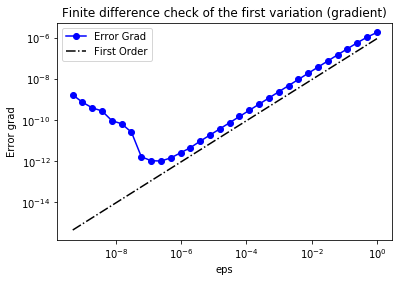### Initial guess

We solve the state equation and compute the cost functional for the initial guess of the parameter m0

m.assign(m0)

# solve state equation
A, state_b = dl.assemble_system (a_state, L_state, bc_state)
dl.solve(A, u.vector(), state_b)

# evaluate cost
[cost_old, misfit_old, reg_old] = cost(u, ud, m, gamma)

# plot
plt.figure(figsize=(15,5))
nb.plot(m,subplot_loc=121, mytitle="m0", vmin=mtrue.vector().min(), vmax=mtrue.vector().max())
nb.plot(u,subplot_loc=122, mytitle="u(m0)")
plt.show()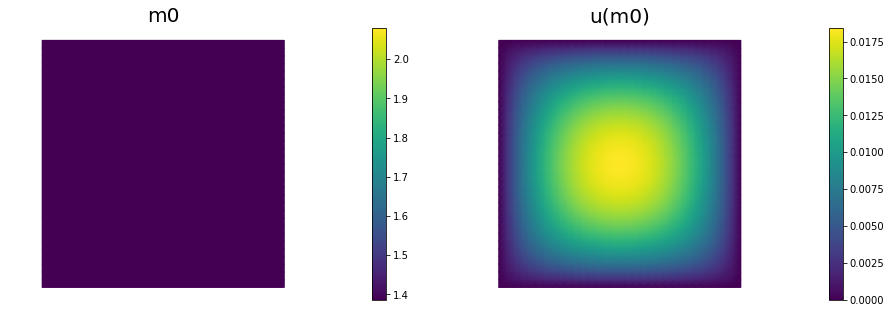We solve the constrained optimization problem using the steepest descent method with Armijo line search.

The stopping criterion is based on a relative reduction of the norm of the gradient (i.e. $\frac{\|g_{n}\|}{\|g_{0}\|} \leq \tau$).

The gradient is computed by solving the state and adjoint equation for the current parameter $m$, and then substituing the current state $u$, parameter $m$ and adjoint $p$ variables in the weak form expression of the gradient:

The Armijo line search uses backtracking to find $\alpha$ such that a sufficient reduction in the cost functional is achieved. Specifically, we use backtracking to find $\alpha$ such that:

# define parameters for the optimization
tol = 1e-4
maxiter = 1000
print_any = 10
plot_any = 50
c_armijo = 1e-5

# initialize iter counters
iter = 0
converged = False

# initializations
g = dl.Vector()
M.init_vector(g,0)

m_prev = dl.Function(Vm)

print( "Nit  cost          misfit        reg         ||grad||       alpha  N backtrack" )

while iter <  maxiter and not converged:

dl.solve(M, g, MG)

# calculate the norm of the gradient

if iter == 0:

# linesearch
it_backtrack = 0
m_prev.assign(m)
alpha = 1.e5
backtrack_converged = False
for it_backtrack in range(20):

m.vector().axpy(-alpha, g )

# solve the state/forward problem
state_A, state_b = dl.assemble_system(a_state, L_state, bc_state)
dl.solve(state_A, u.vector(), state_b)

# evaluate cost
[cost_new, misfit_new, reg_new] = cost(u, ud, m, gamma)

# check if Armijo conditions are satisfied
if cost_new < cost_old - alpha * c_armijo * grad_norm2:
cost_old = cost_new
backtrack_converged = True
break
else:
alpha *= 0.5
m.assign(m_prev)  # reset a

if backtrack_converged == False:
converged = False
break

sp = ""
if (iter % print_any)== 0 :
print( "%3d %1s %8.5e %1s %8.5e %1s %8.5e %1s %8.5e %1s %8.5e %1s %3d" % \
(iter, sp, cost_new, sp, misfit_new, sp, reg_new, sp, \
gradnorm, sp, alpha, sp, it_backtrack) )

if (iter % plot_any)== 0 :
nb.multi1_plot([m,u,p], ["m","u","p"], same_colorbar=False)
plt.show()

# check for convergence
converged = True
print ("Steepest descent converged in ",iter,"  iterations")

iter += 1

if not converged:
print ( "Steepest descent did not converge in ", maxiter, " iterations")

Nit  cost          misfit        reg         ||grad||       alpha  N backtrack
0   1.11658e-05   1.10372e-05   1.28638e-07   6.09224e-05   5.00000e+04     1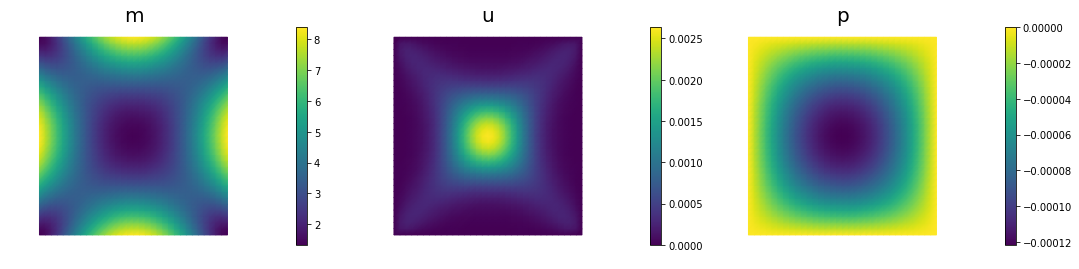10   3.07468e-06   2.81411e-06   2.60567e-07   9.96798e-06   2.50000e+04     2
20   1.68333e-06   1.36733e-06   3.15999e-07   4.79045e-06   2.50000e+04     2
30   1.08642e-06   7.90406e-07   2.96014e-07   1.08801e-06   1.00000e+05     0
40   6.99077e-07   4.72703e-07   2.26374e-07   2.65053e-06   2.50000e+04     2
50   4.33194e-07   2.77234e-07   1.55960e-07   1.89540e-06   2.50000e+04     2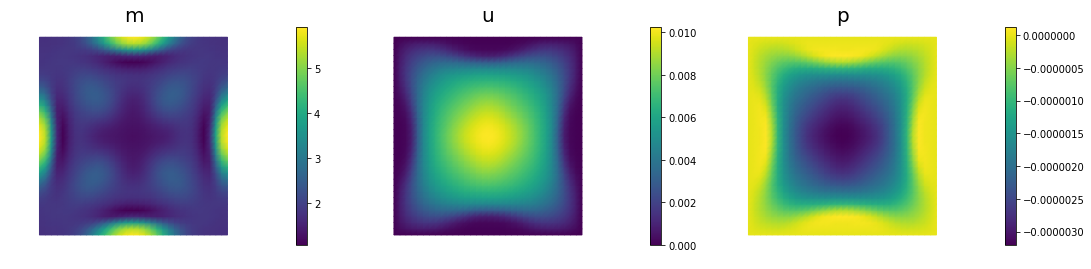60   2.08625e-07   1.30743e-07   7.78817e-08   2.16983e-06   2.50000e+04     2
70   6.76060e-08   4.57894e-08   2.18166e-08   8.24665e-07   5.00000e+04     1
80   1.48917e-08   1.10392e-08   3.85253e-09   6.15229e-07   5.00000e+04     1
90   6.29351e-09   4.15392e-09   2.13959e-09   1.56127e-07   5.00000e+04     1
100   5.60737e-09   3.69792e-09   1.90946e-09   4.43594e-08   5.00000e+04     1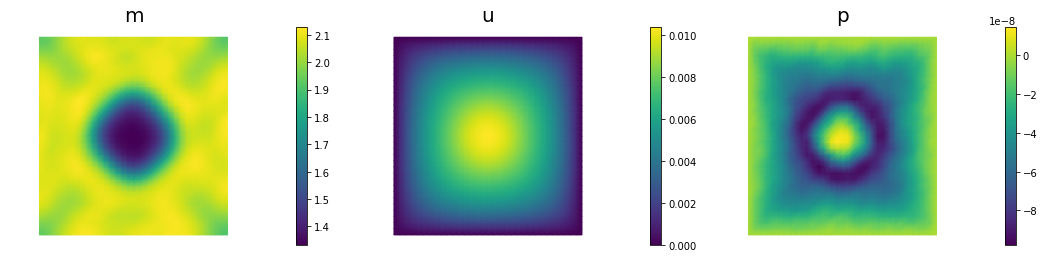110   5.50313e-09   3.68332e-09   1.81981e-09   1.54409e-08   5.00000e+04     1
120   5.46016e-09   3.69724e-09   1.76293e-09   1.25966e-08   5.00000e+04     1
130   5.43430e-09   3.70647e-09   1.72783e-09   1.07081e-08   5.00000e+04     1
140   5.41730e-09   3.71189e-09   1.70542e-09   9.20452e-09   5.00000e+04     1
150   5.40583e-09   3.71517e-09   1.69066e-09   7.93538e-09   5.00000e+04     1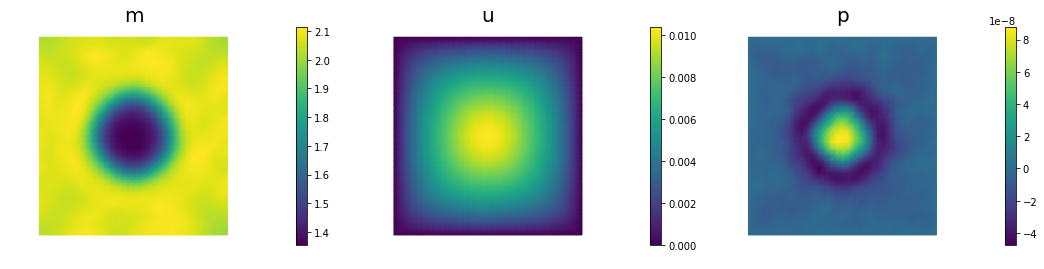Steepest descent converged in  153   iterations

nb.multi1_plot([mtrue, m], ["mtrue", "m"])
nb.multi1_plot([u,p], ["u","p"], same_colorbar=False)
plt.show()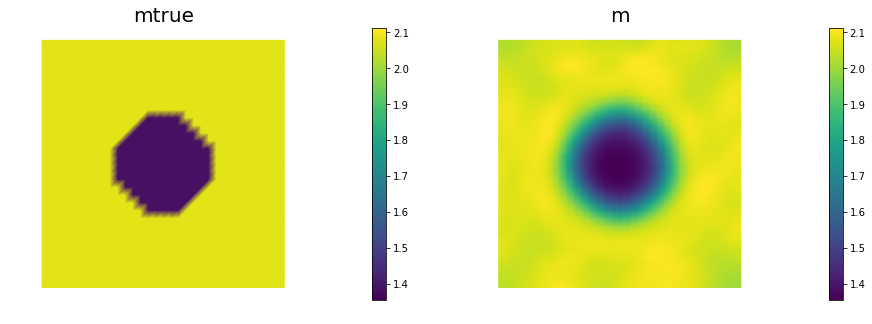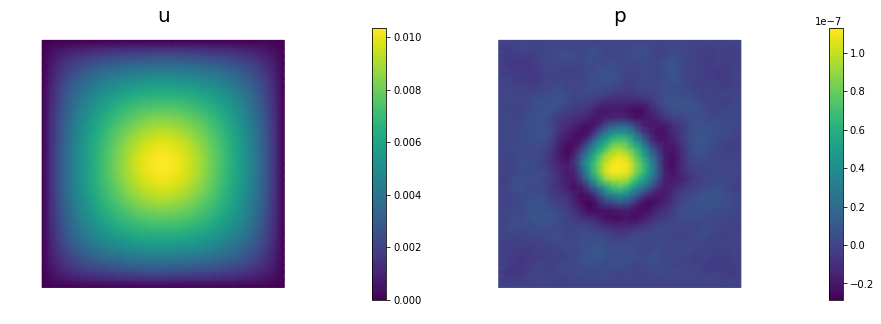## Hands on

### Question 1

Report the number of steepest descent iterations for a discretization of the domain with $8 \times 8$, $16 \times 16$, $32 \times 32$, $64 \times 64$ finite elements and give the number of unknowns used to discretize the log diffusivity field m for each of these meshes. Discuss how the number of iterations changes as the inversion parameter mesh is refined, i.e., as the parameter dimension increases. Is steepest descent method scalable with respect to the parameter dimension?

The number of interations increases as we refine the mesh. Steepest descent method is not scalable with respect to the parameter dimension.

### Question 2

Add the advective term $\mathbf{v} = [30,0]^t$ to the inverse problem and its hIPPYlib/FEniCS implementation and plot the resulting reconstruction of $m$ for a noise level of 0.01 and for a reasonably chosen regularization parameter.

See function def AddDiffInverseProblem(nx, ny, v, gamma, useTV, TVeps, plotting) for the implementation. Using Morozov’s discrepancy principle we chose the regularization parameter $\gamma = 10^{-9}$.

### Question 3

Since the coefficient $m$ is discontinuous, a better choice of regularization is total variation rather than Tikhonov regularization, to prevent an overly smooth reconstruction. Modify the implementation and plot the result for a reasonably chosen regularization parameter $\gamma$. Use $\varepsilon = 0.1$ to make the non-differentiable TV regularization term differentiable ($\varepsilon = 0.1$ has the same meaning as in Lab 2). In other words, your regularization functional should be:

See function def AddDiffInverseProblem(nx, ny, v, gamma, useTV, TVeps, plotting) for the implementation. Using Morozov’s discrepancy principle we choose the regularization parameter $\gamma = 10^{-8}$.

def AddDiffInverseProblem(nx, ny, v, gamma, useTV=False, TVeps=None, plotting=False):
np.random.seed(seed=1)
mesh = dl.UnitSquareMesh(nx, ny)
Vm = dl.FunctionSpace(mesh, 'Lagrange', 1)
Vu = dl.FunctionSpace(mesh, 'Lagrange', 2)

# The true and initial guess for inverted parameter
mtrue = dl.interpolate(
dl.Expression('std::log( 8. - 4.*(pow(x - 0.5,2) + pow(x - 0.5,2) < pow(0.2,2) ) )', degree=5),
Vm)

# define function for state and adjoint
u = dl.Function(Vu)
m = dl.Function(Vm)
p = dl.Function(Vu)

# define Trial and Test Functions
u_trial, m_trial, p_trial = dl.TrialFunction(Vu), dl.TrialFunction(Vm), dl.TrialFunction(Vu)
u_test,  m_test,  p_test  = dl.TestFunction(Vu),  dl.TestFunction(Vm),  dl.TestFunction(Vu)

# initialize input functions
f  = dl.Constant(1.0)
u0 = dl.Constant(0.0)

# set up dirichlet boundary conditions
def boundary(x,on_boundary):
return on_boundary

bc_state = dl.DirichletBC(Vu, u0, boundary)

# noise level
noise_level = 0.01

# weak form for setting up the synthetic observations
L_true = f * u_test * dl.dx

# solve the forward/state problem to generate synthetic observations
A_true, b_true = dl.assemble_system(a_true, L_true, bc_state)

utrue = dl.Function(Vu)
dl.solve(A_true, utrue.vector(), b_true)

ud = dl.Function(Vu)
ud.assign(utrue)

# perturb state solution and create synthetic measurements ud
# ud = u + ||u||/SNR * random.normal
MAX = ud.vector().norm("linf")
noise = dl.Vector()
A_true.init_vector(noise,1)
noise.set_local( noise_level * MAX * np.random.normal(0, 1, len(ud.vector().get_local())) )

ud.vector().axpy(1., noise)

if plotting:
nb.multi1_plot([utrue, ud], ["State solution with mtrue", "Synthetic observations"])
plt.show()

# Define cost function
def cost(u, ud, m, gamma):
if useTV:
else:

misfit = 0.5 * dl.assemble( (u-ud)**2*dl.dx)
return [reg + misfit, misfit, reg]

# weak form for setting up the state equation
L_state = f * u_test * dl.dx

# weak form for setting up the adjoint equations
L_adj = - dl.inner(u - ud, p_test) * dl.dx

if useTV:
else:

# Mass matrix in parameter space
Mvarf    = dl.inner(m_trial, m_test) * dl.dx
M = dl.assemble(Mvarf)

m0 = dl.interpolate(dl.Constant(np.log(4.) ), Vm )

m.assign(m0)

# solve state equation
A, state_b = dl.assemble_system (a_state, L_state, bc_state)
dl.solve(A, u.vector(), state_b)

# evaluate cost
[cost_old, misfit_old, reg_old] = cost(u, ud, m, gamma)

if plotting:
plt.figure(figsize=(15,5))
nb.plot(m,subplot_loc=121, mytitle="m0", vmin=mtrue.vector().min(), vmax=mtrue.vector().max())
nb.plot(u,subplot_loc=122, mytitle="u(m0)")
plt.show()

tol = 1e-4
maxiter = 1000
c_armijo = 1e-5

# initialize iter counters
iter = 0
converged = False

# initializations
g = dl.Vector()
M.init_vector(g,0)

m_prev = dl.Function(Vm)

while iter <  maxiter and not converged:

dl.solve(M, g, MG)

# calculate the norm of the gradient

if iter == 0:

# linesearch
it_backtrack = 0
m_prev.assign(m)
alpha = 1.e5
backtrack_converged = False
for it_backtrack in range(20):

m.vector().axpy(-alpha, g )

# solve the state/forward problem
state_A, state_b = dl.assemble_system(a_state, L_state, bc_state)
dl.solve(state_A, u.vector(), state_b)

# evaluate cost
[cost_new, misfit_new, reg_new] = cost(u, ud, m, gamma)

# check if Armijo conditions are satisfied
if cost_new < cost_old - alpha * c_armijo * grad_norm2:
cost_old = cost_new
backtrack_converged = True
break
else:
alpha *= 0.5
m.assign(m_prev)  # reset a

if backtrack_converged == False:
converged = False
break

# check for convergence
converged = True
print ("Steepest descent converged in ",iter,"  iterations")

iter += 1

if not converged:
print ( "Steepest descent did not converge in ", maxiter, " iterations")

if plotting:
nb.multi1_plot([mtrue, m], ["mtrue", "m"])
nb.multi1_plot([u,p], ["u","p"], same_colorbar=False)
plt.show()

Mstate = dl.assemble(u_trial*u_test*dl.dx)
noise_norm2 = noise.inner(Mstate*noise)
return Vm.dim(), iter, noise_norm2, cost_new, misfit_new, reg_new

## Question 1
ns = [8,16,32, 64]
niters = []
ndofs  = []

for n in ns:
ndof, niter, _,_,_,_ = AddDiffInverseProblem(nx=n, ny=n, v=dl.Constant((0., 0.)),
gamma = 1e-9, useTV=False, plotting=False)
niters.append(niter)
ndofs.append(ndof)

plt.semilogx(ndofs, niters)
plt.ylim([0, 1000])
plt.xlabel("Parameter dimension")
plt.ylabel("Number of iterations")

Steepest descent converged in  135   iterations
Steepest descent converged in  143   iterations
Steepest descent converged in  153   iterations
Steepest descent converged in  357   iterations

Text(0,0.5,'Number of iterations')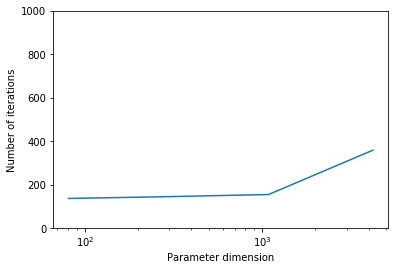## Question 2
n = 16
gammas = [1e-7, 1e-8, 1e-9, 1e-10]
misfits = []

for gamma in gammas:
ndof, niter, noise_norm2, cost,misfit,reg = AddDiffInverseProblem(nx=n, ny=n, v=dl.Constant((30., 0.)),
gamma = gamma, useTV=False, plotting=False)
misfits.append(misfit)

plt.loglog(gammas, misfits, "-*b", label="Misfit")
plt.loglog([gammas,gammas[-1]], [.5*noise_norm2, .5*noise_norm2], "-r", label="Squared norm noise")
plt.title("Morozov discrepancy principle")
plt.xlabel("Regularization parameter")
plt.ylabel("Misfit cost")
plt.show()

print("Solve for gamma = ", 1e-9)
_ = AddDiffInverseProblem(nx=n, ny=n, v=dl.Constant((30., 0.)), gamma = 1e-8, useTV=False, plotting=True)

Steepest descent converged in  233   iterations
Steepest descent converged in  154   iterations
Steepest descent converged in  139   iterations
Steepest descent converged in  256   iterations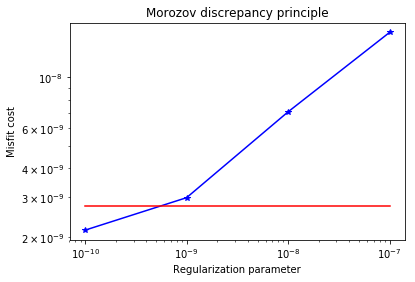Solve for gamma =  1e-09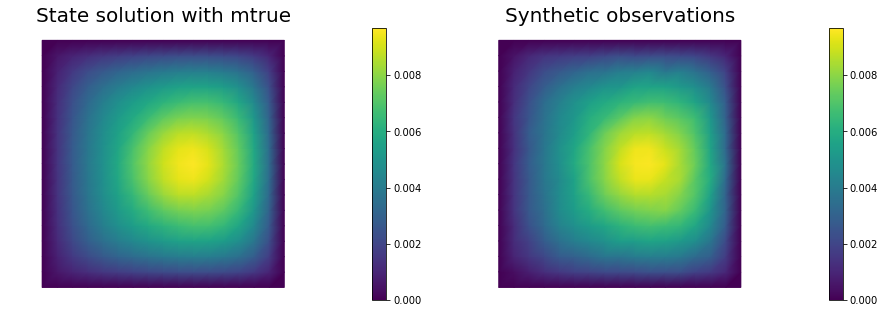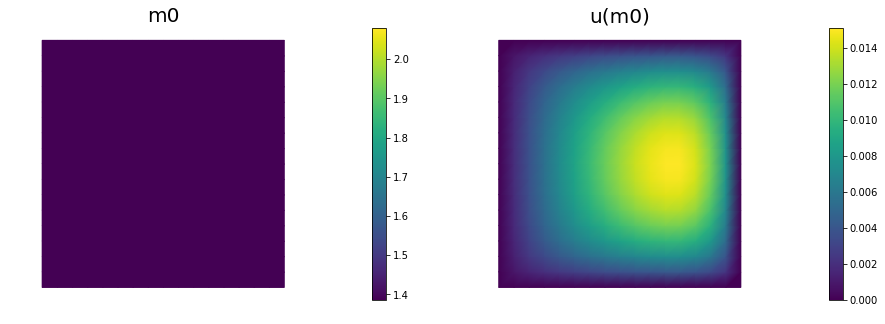Steepest descent converged in  154   iterations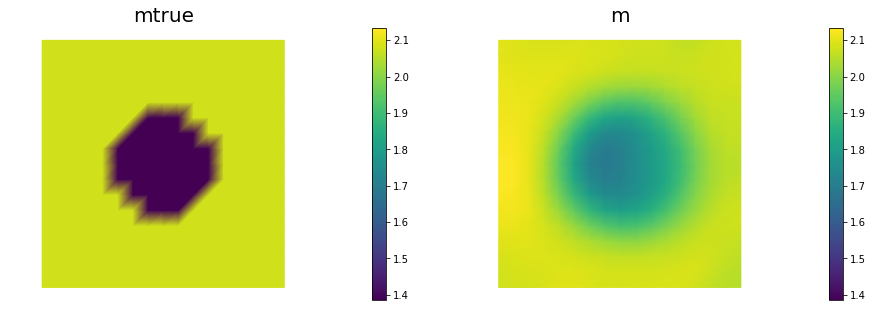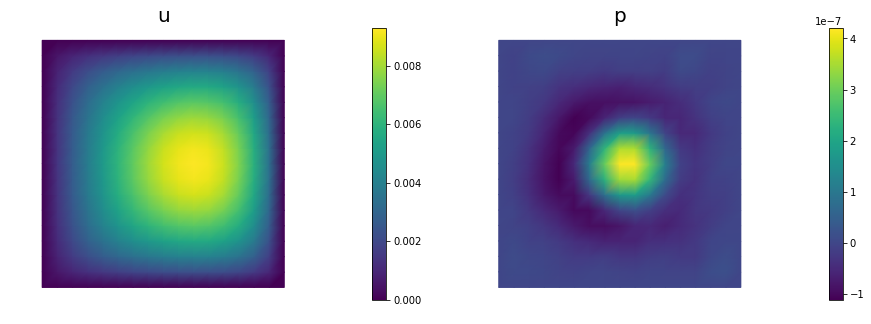## Question 3
n = 16
gammas = [1e-7, 1e-8, 1e-9, 1e-10]
misfits = []

for gamma in gammas:
ndof, niter, noise_norm2, cost,misfit,reg = AddDiffInverseProblem(nx=n, ny=n, v=dl.Constant((30., 0.)),
gamma = gamma, useTV=True, TVeps=dl.Constant(0.1), plotting=False)
misfits.append(misfit)

plt.loglog(gammas, misfits, "-*b", label="Misfit")
plt.loglog([gammas,gammas[-1]], [.5*noise_norm2, .5*noise_norm2], "-r", label="Squared norm noise")
plt.title("Morozov discrepancy principle")
plt.xlabel("Regularization parameter")
plt.ylabel("Misfit cost")
plt.show()

print("Solve for gamma = ", 1e-8)
_ = AddDiffInverseProblem(nx=n, ny=n, v=dl.Constant((30., 0.)), gamma = 1e-8, useTV=True,
TVeps=dl.Constant(0.1), plotting=True)

Steepest descent converged in  928   iterations
Steepest descent converged in  376   iterations
Steepest descent converged in  192   iterations
Steepest descent converged in  267   iterations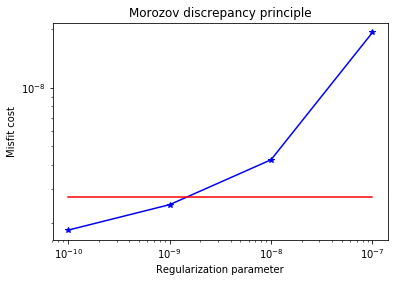Solve for gamma =  1e-08Steepest descent converged in  376   iterations